﻿ Radiometric Cross-Calibration for Multiple Sensors with the Moon as an Intermediate Reference
 J. Meteor. Res.2019, Vol. 33Issue (5): 925-933PDF
http://dx.doi.org/10.1007/s13351-019-9008-y
The Chinese Meteorological Society
0

#### Article Information

ZHANG, Lu, Peng ZHANG, Xiuqing HU, et al., 2019.
Radiometric Cross-Calibration for Multiple Sensors with the Moon as an Intermediate Reference. 2019.
J. Meteor. Res., 33(5): 925-933
http://dx.doi.org/10.1007/s13351-019-9008-y

### Article History

in final form July 15, 2019
Radiometric Cross-Calibration for Multiple Sensors with the Moon as an Intermediate Reference
Lu ZHANG1,2,3, Peng ZHANG3, Xiuqing HU3, Lin CHEN3, Min MIN3, Na XU3, Ronghua WU3
1. Nanjing University of Information Science & Technology, Nanjing 210044;
3. Key Laboratory of Radiometric Calibration and Validation for Environmental Satellites, National Satellite Meteorological Center, China Meteorological Administration, Beijing 100081
ABSTRACT: The instrument cross-calibration is an effective way to assess the quality of satellite data. In this study, a new me-thod is proposed to cross-calibrate the sensors among satellite instruments by using a RObotic Lunar Observatory (ROLO) model and Apollo sample reflectance in reflective solar bands (RSBs). The ROLO model acts as a transfer radiometer to bridge between the instruments. The reflective spectrum of the Apollo sample is used to compensate for the difference in the instrument’s relative spectral responses (RSRs). In addition, the double ratio between the observed lunar irradiance and the simulated lunar irradiance is used to reduce the difference in instrument lunar viewing and illumining geometry. This approach is applied to the Moderate Resolution Imaging Spectroradiometer (MODIS), the Sea-Viewing Wide Field-of-View Sensor (SeaWiFS), and the Advanced Land Imager (ALI) on board three satellites, respectively. The mean difference between MODIS and SeaWiFS is less than 3.14%, and the difference between MODIS and ALI is less than 4.75%. These results indicate that the proposed cross-calibration method not only compensates for the RSR mismatches but also reduces the differences in lunar observation geometry. Thus, radiance calibration of any satellite instrument can be validated with a reference instrument bridged by the moon.
Key words: radiometric cross-calibration     multiple sensors     calibration reference
1 Introduction

For satellites without onboard calibration systems, the instrument can be calibrated through comparing with other reference instruments. This technique is typically referred to as cross-calibration. For solar reflective bands, the cross-calibration processing must consider different relative spectral responses (RSRs), different viewing, or illuminating geometries of the target, and many other factors (Kieffer and Stone, 2005; Chander et al., 2013a). Chander et al. (2013b) grouped the methods and measurements involved in sensor radiometric cross-calibration into a number of categories. In this paper, we focus on radiometric cross-calibration using lunar observations.

A change of the lunar irradiance is about 1% in a 1.4-giga year (Gyr) period (Kieffer, 1997). The moon is neither strongly colored nor strongly variegated in color, and it is surrounded by a black field (Kieffer, 1997) and is thus a suitable candidate for use in calibration. There are two popularly used lunar irradiance models: the RObotic Lunar Observatory (ROLO) model and the Miller–Turner (2009) (MT2009) model (Miller and Turner, 2009)). The ROLO model was developed by the United States Geological Survey and was used as a standard for calibration in the wavelength ranging from 350 to 2500 nm. The MT2009 used the moon as a source of visible radiation for nighttime sensing of the earth’s atmosphere and surface. It covered 202–2800 nm (Kieffer and Stone, 2005; Miller and Turner, 2009). These two models express the lunar reflectance as a function of viewing and illumination geometry, and the uncertainties of ROLO (311g version) model and MT2009 are 5%–10% and 7%–15%, respectively (Kieffer and Stone, 2005; Miller and Turner, 2009; Shao et al., 2014). Since it is difficult to use the current lunar model as an absolute calibrator, the lunar model is commonly used as an intermediate reference for cross-calibration (Wang et al., 2011).

For the purpose of cross-calibration using the moon, instruments should measure the lunar radiation from the same viewing and illumination geometry with the same RSRs. However, these idealized conditions are rarely satisfied for a pair of instruments. Therefore, some methods are proposed to reduce the calibration error sources. Sun et al. (2003) showed that normalized lunar irradiance measured with a Moderate Resolution Imaging Spectroradiometer (MODIS) can provide reliable monitoring of radiometric stability over the long term. Xiong et al. (2008) performed an intercomparison between Terra and Aqua MODIS reflective solar bands (RSBs) to monitor the on-orbit calibration consistency by using lunar view data. Eplee et al. (2011) performed a cross-calibration of the MODIS and Sea-Viewing Wide Field-of-View Sensor (SeaWiFS) based on the ROLO model. Shao et al. (2014) compared the lunar irradiance of the MT2009 model and a Geostationary Operational Environmental Satellite-R Series (GOES-R) Advanced Baseline Imager (ABI). The double difference method can relax the requirements in the viewing and illumination geometry and allow for indirect comparisons over a much wider range of conditions (Chander et al., 2013c).

In this study, a lunar calibration method is proposed to cross-calibrate the sensors among satellite instruments. The ROLO model is used as an intermediate reference to bridge the instrument calibration. To reduce the errors from the difference of RSRs and the lunar observation geometry, we use the reflective spectrum of the Apollo sample to compensate for the difference in the instrument RSRs and the double ratio between the observed and the simulated lunar irradiance.

The paper is organized as follows. Section 2 presents a method of spectral band adjustment (SBA) based on the scaled reflectance of the Apollo sample to reduce the cross-calibration uncertainties by the discrepancy in the RSRs in the RSBs. The SeaWiFS and MODIS lunar observation with nearly the same phase angles are selected to check the SBA method based on the reflectance of the Apollo samples in the RSBs. In Section 3, the double ratio and double difference methods are compared in the MODIS RSBs to reduce the impacts from the lunar viewing and illumining geometry results. In Section 4, the proposed lunar calibration method is applied to MODIS and SeaWiFS as well as MODIS and Advanced Land Imager (ALI), respectively.

2 Spectral band adjustments using scaled lunar reflectance 2.1 RSRs

The instrument on board a satellite has its spectral coverage and RSRs required for the observations of earth. MODIS, SeaWiFS, and ALI’s RSRs at visible and near-infrared wavelength regions are shown in Fig. 1. The SBA of RSR has been used for cross-calibration in past many years. For example, Teillet et al. (2001) used a reference hyperspectral to cross-calibrate Advanced Very High-Resolution Radiometer (AVHRR) onboard NOAA-14, SeaWiFS, and the Thematic Mapper (TM) onboard Landsat-5 with different RSRs. Teillet et al. (2007) investigated the effects of the spectral band difference on the radiometric cross-calibration between satellite sensors, using the near-simultaneous overpassing of common ground targets. Cao et al. (2007) showed the discrepancy in RSRs between MODIS and AVHRR bands at the simultaneous nadir overpasses (SNO) in polar regions. Chander et al. (2013a) used their near-simultaneous observations (within 30 min) to estimate the SBA factors. Henry et al. (2013) collected hyperspectral reflectances from the desert as measured by Hyperion and used them to reduce the bias of the RSRs and to cross-calibrate the Enhanced Thematic Mapper (ETM+) onboard Landsat-7, Terra MODIS, Aqua MODIS, and Environmental Satellite MEdium Resolution Imaging Spectrometer (MERIS).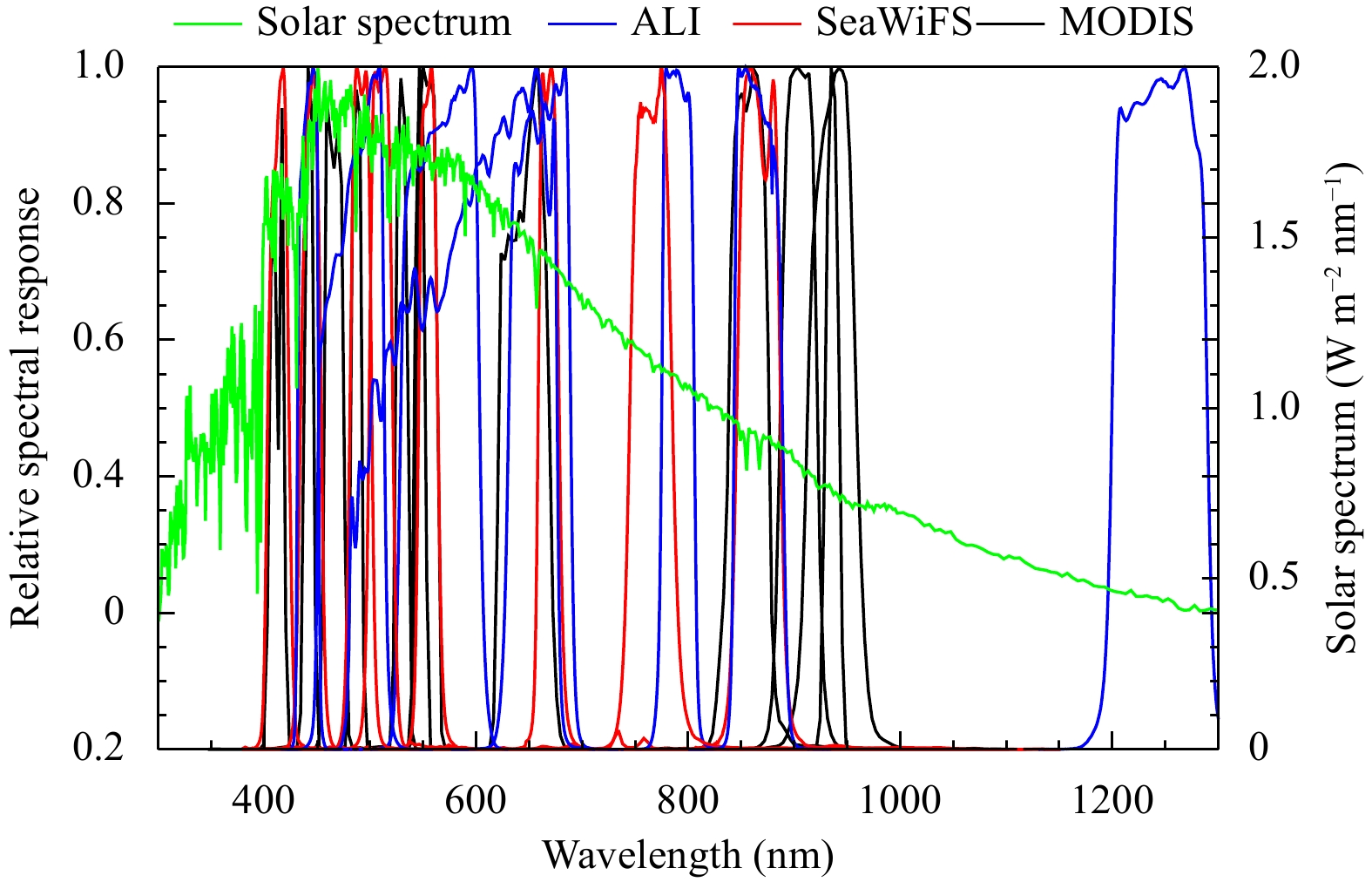Figure 1 A portion of the RSRs from the ALI, MODIS, SeaWiFS, and solar spectrum.

It is difficult to do the cross-calibration for a pair of instruments that have a discrepancy in their RSRs. Here, a method is developed to reduce the error in the cross-calibration.

2.2 Lunar reflectance

Pieters et al. (2013) gathered samples of lunar radiance and found that the radiance of Serenitatis and Messier A are not strongly colored or strongly variegated. The lunar reflectance measured by shortwave near-infrared Grating Spectrometer (SIR-2), Moon Mineralogy Mapper (M3) on Chandrayaan-1, Spectral Profiler (SP), and Multiband Imager (MI) on SELENE (SELenological and ENgineering Explorer) also show that the reflectance of the lunar surface is smooth (Ohtake et al., 2013; Coman et al., 2018). As a result, a selected lunar reflective spectrum from the mixed Apollo samples is used in this paper. They include Apollo samples of soil (sample 62231) and breccia (sample 67455). The mixed Apollo is 95% soil and 5% breccia (Kieffe and Stone, 2005).

2.3 The methodology for SBA

To perform the SBA for the different sensors, we propose satellite A as the radiometric reference while satellite B acts as the satellite that is in need of calibration. Four major steps are involved in the cross-calibration of sensors with different RSRs: 1) the lunar irradiance of satellite A is converted to the lunar effective disk reflectance; 2) the reflective spectrum of Apollo samples is scaled by the lunar effective disk reflectance of satellite A; 3) the scaled reflective spectrum is convoluted with the RSRs of satellite B; and 4) the lunar reflectance of satellite B is converted to the lunar irradiance of satellite B. As a result, the sensors’ RSR differences are rectified. The details of the four steps are shown in the following flowchart (Fig. 2).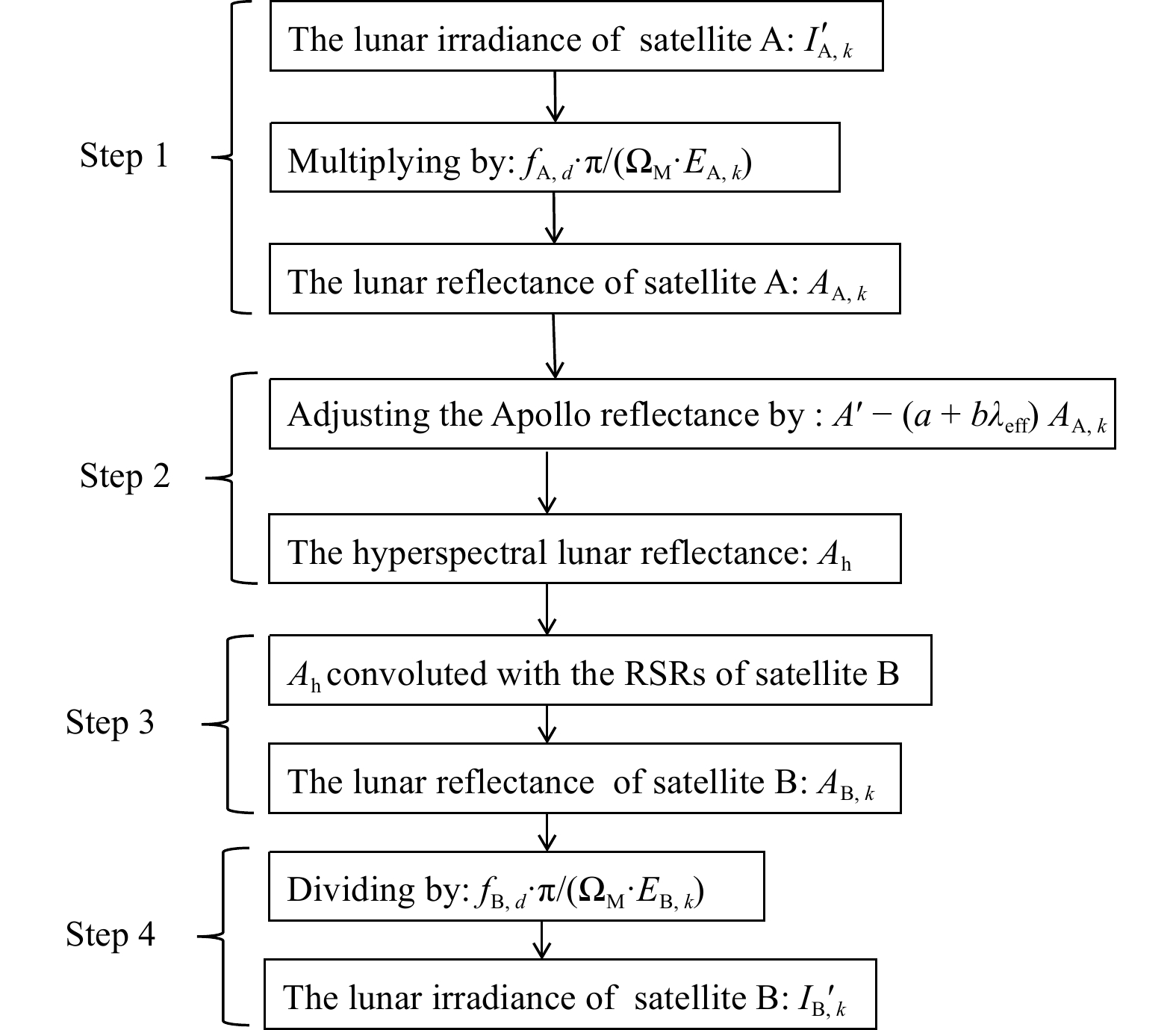Figure 2 Flowchart for the spectral band adjustment (SBA) of RSRs using Apollo sample reflectance.

1) The lunar effective disk reflectance of satellite A. As described by Eq. (1), ${A_{A,k}}$ is satellite A’s effective disk equivalent reflectance for band k at the standard distance. The standard distance between the sun and earth is 1 AU, and the standard distance between satellite A and the moon is 384,400 km (Kieffer and Stone, 2005). The conversion between the irradiance and the effective disk equivalent reflectance is expressed as follows:

 ${A_{{\rm A},k}} = {I'_{{\rm A},k}} \cdot {f_{{\rm A},d}} \cdot \pi /\left({{\Omega _{\rm M}} \cdot {E_{{\rm A},k}}} \right),$ (1)

where ${f_{{\rm A},d}} = {\left({\displaystyle\frac{{{D_{{\rm S} - {\rm L}}}}}{{1{\rm{AU}}}}} \right)^2}{\left({\displaystyle\frac{{{D_{{\rm A} - {\rm L}}}}}{{384400\;{\rm{km}}}}} \right)^2}$ , ${I'_{A,k}}$ is the satellite A’s lunar irradiance at band k, $\Omega _ {\rm M}$ is the solid angle of the moon (6.4177 × 10−5 sr), ${D_{{\rm S} - {\rm L}}}$ is the distance between the sun and moon, and ${D_{{\rm A} - {\rm L}}}$ is the distance between satellite A and the moon. ${E_{{\rm A},k}}$ is the solar spectral irradiance of satellite A at bandk as follows:

 ${E_{{\rm A},k}}=\frac{{\int_{{\lambda _2}}^{{\lambda _1}} {{\rm{ir}}{{\rm{r}}_{\rm solar}}\left(\lambda \right) \cdot {\rm{RS}}{{\rm{R}}_{{\rm{A,}}k}}\left(\lambda \right) {\rm d} \lambda } }}{{\int_{{\lambda _2}}^{{\lambda _1}} {{\rm RS}{{\rm R}_{{\rm{A,}}k}}\left(\lambda \right) {\rm d} \lambda } }},$ (2)

where ${\rm{RS}}{{\rm{R}}_{{\rm A},k}}\left(\lambda \right)$ is the RSR of satellite A at band kand ${\rm{ir}}{{\rm{r}}_{{\rm{solar}}}}\left(\lambda \right)$ is the solar irradiance of the spectrum.

2) The lunar reflective spectrum scaled by satellite A. The mixed Apollo reflectance $A'$ (95% soil and 5% breccia) is scaled to the effective disk reflectance of satellite A with a linear function of the wavelength as follows:

 $A' = \left({a + b{\lambda _{{\rm{eff}}}}} \right){A_{{\rm A},k}},$ (3)

where ${\lambda _{{\rm{eff}}}}$ is the effective wavelength of satellite A shown in Eq. (4), ${\lambda _1}$ is the upper limit of RSR’s wavelength, ${\lambda _2}$ is the lower limit of RSR’s wavelength, and $a$ , $b$ are the fitting parameters:

 ${\lambda _{\rm eff}} = \frac{{\int_{{\lambda _2}}^{{\lambda _1}} {\lambda \cdot {\rm{RS}}{{\rm{R}}_{{\rm A},k}}\left(\lambda \right) {\rm d} \lambda } }}{{\int_{{\lambda _2}}^{{\lambda _1}} {{\rm{RS}}{{\rm{R}}_{{\rm A},k}}\left(\lambda \right) {\rm d} \lambda } }},$ (4)

note that $a$ and $b$ are deduced by the least squares fitting of Eq. (3). Thus, the lunar effective disk reflectance of satellite A is upgraded to hyperspectral reflectance ${A_{\rm h}}$ as follows:

 ${A_{\rm h}} = \frac{{A'}}{{\left({a + b{\lambda _{\rm h}}} \right)}},$ (5)

where ${\lambda _{\rm h}}$ is the wavelength of the mixed Apollo reflectance.

3) The scaled reflective spectrum is convoluted with the RSRs of satellite B. The hyperspectral reflectance is convoluted with the RSRs of satellite B as follows:

 ${A_{{\rm B},k}}=\frac{{\int_{{\lambda _2}}^{{\lambda _1}} {{A_{\rm h}}} \cdot {\rm{RS}}{{\rm{R}}_{{\rm B},k}}\left( \lambda \right){\rm d}\lambda } }{{\int_{{\lambda _2}}^{{\lambda _1}} {{\rm{RS}}{{\rm{R}}_{{\rm B},k}}\left( \lambda \right){\rm d}\lambda } }},$ (6)

where ${\rm{RS}}{{\rm{R}}_{{\rm B},k}}\left(\lambda \right)$ is the RSR of satellite B at band k.

4) The lunar irradiance of satellite B. By reversing step (1), we can obtain lunar irradiance ${I'_{{\rm{B}},k}}$ of satellite B at band k. Therefore, the different RSRs between satellites A and B are compensated by the mixed Apollo reflectance.

2.4 Evaluating the SBA results

TheAqua MODIS is commonly referred to as a standard instrument, and it is an ideal for cross-calibration. The accepted radiometric calibration uncertainty forAqua MODIS is 2%–3% (Xiong et al., 2003, 2007, 2016). The MODIS acquires lunar measurements in the reflective solar spectrum from 0.41 to 2.1 µm with its narrow RSRs. The key onboard calibrators associated with the RSBs are the solar diffuser (SD) and the solar diffuser stability monitor (SDSM). Regular SD observations, coupled with periodic SDSM measurements from desert, and Antarctic sites, deep convective clouds (DCC) and lunar measurements, help to characterize the individual detector gain for MODIS RSBs (Xiong et al., 2007, 2016; Angal et al., 2016). Thus, the AquaMODIS is selected as a quasi-standard tool for evaluating the cross-calibration bias of the other satellites.

The radiometric stability of SeaWiFS in orbit also displays a good performance. The NASA Ocean Biology Processing Group’s Calibration and Validation Team noted that the on-orbit radiometric stability of SeaWiFS is 0.1% over the 9-yr mission. The accuracy (biases in the measurements) of the SeaWiFS is 2%–3% from lunar calibration and 1%–2% from vicarious calibration (Barnes et al., 2001; Eplee et al., 2011, 2012). Therefore, the SeaWiFS is used for comparisons with the MODIS.

Stone (2008) compared the SeaWiFS and MODIS lunar irradiance with similar phase angles. The results are shown in Table 1. In their study, the different RSRs of SeaWiFS and MODIS are not considered. To compensate for the RSR impacts on the cross-calibration, the SBA by the scaled Apollo sample reflectance mentioned above is used to reduce the error from different RSRs of SeaWiFS and MODIS. A similar phase angle is selected to minimize the impact from the observation geometry.

Table 1 The lunar measurements for MODIS and SeaWiFS (Stone, 2008)
 Observation geometry (degree) Time Phase Sublunar longitude Sublunar latitude Distance correction SeaWiFS 2006-12-09T08:27:51Z 54.49 6.39 −4.29 0.99 MODIS 2006-12-09T09:34:11Z 55.12 6.47 −3.62 1.01 Lunar irradiance (μW m−2 nm−1) SeaWiFS band 1 2 3 4 5 6 7 8 Wavelength (nm) 414.20 444.10 491.90 510.30 556.60 668.50 767.80 864.90 MODIS band 8 9 10 4 13 16 Wavelength (nm) 414.90 443.30 487.30 554.40 670.30 866.60 Observation time: 2006-12-09 SeaWiFS 0.728 0.903 1.073 1.078 1.169 1.170 1.067 0.861 Model 0.732 0.890 1.025 1.037 1.115 1.102 0.984 0.830 MODIS 0.766 0.908 1.063 1.154 1.134 0.862 Model 0.708 0.844 0.970 1.077 1.063 0.795

As described in Section 2.3, MODIS lunar irradiance, ${I'_k}$ is converted to the lunar reflectance ${A_k}$ . The equation ${A'_k} = \left({a + b\lambda } \right){A_k}$ is then used to generate the adjusted lunar reflective spectrum (the dashed red line in Fig. 3) by using the MODIS lunar reflectance from the original Apollo sample reflective spectrum (the solid red line in Fig. 3). Finally, the MODIS band reflectance after SBA for SeaWiFS (the simulated SeaWiFS bands reflectance) can be obtained from the scaled lunar reflective spectrum convoluted with the SeaWiFS’s RSRs (the black diamond in Fig. 3).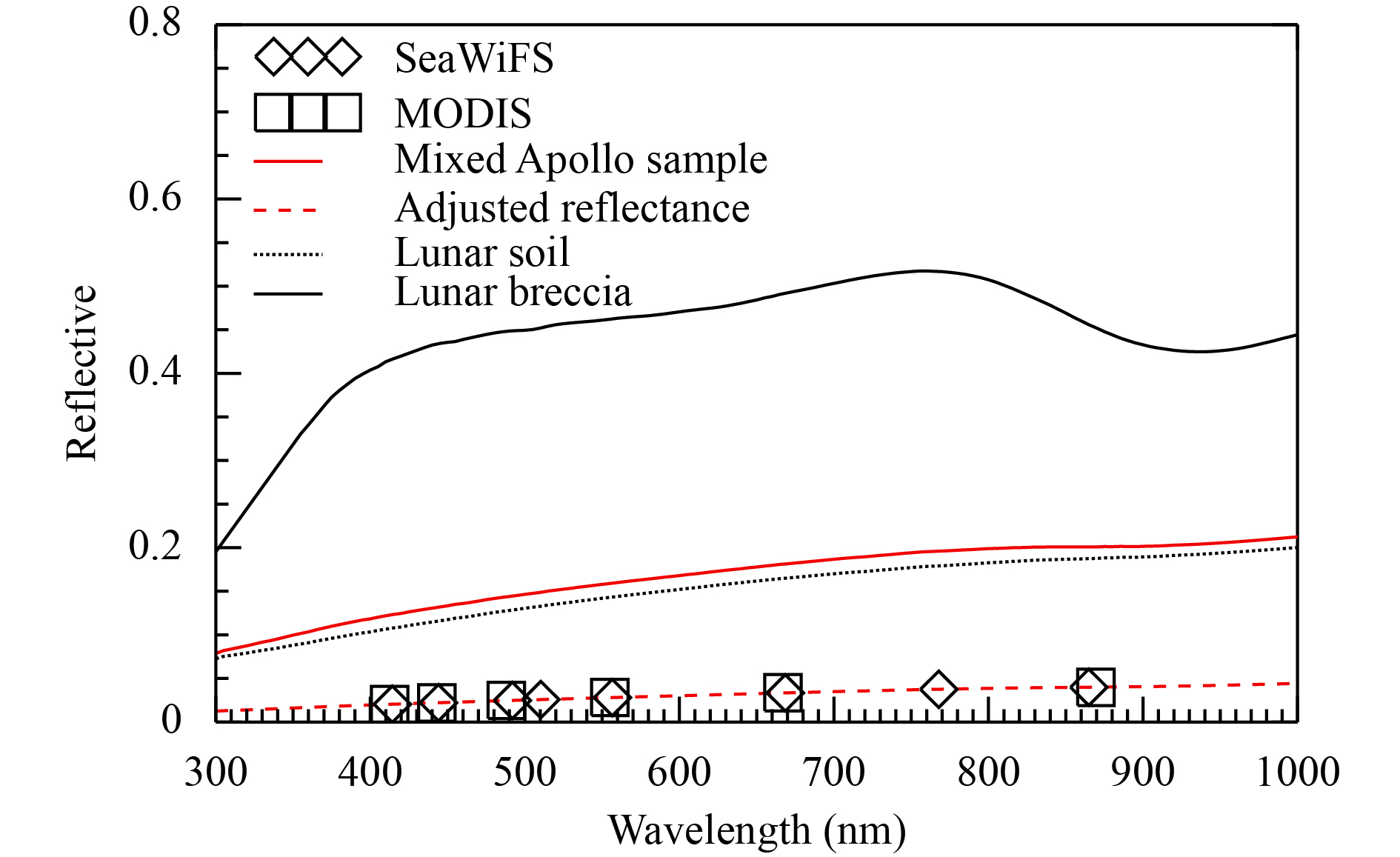Figure 3 The adjusted lunar reflective spectrum.

The reflectance difference between the MODIS and SeaWiFS with and without an SBA is shown in Fig. 4. The black dashed line with asterisks indicates a direct comparison between the SeaWiFS and MODIS without the SBA, and the black solid line with rectangles corresponds a comparison with the SBA. Except for bands 2 and 8 of the SeaWiFS, the reflectance difference between these two instruments has been clearly reduced with the SBA. This method can also be used to simulate the new channels, which the reference instrument did not cover. For example, SeaWiFS bands 4 (410.3 nm) and 7 (767.80 nm) were not covered by the MODIS (red rectangle in Fig. 4).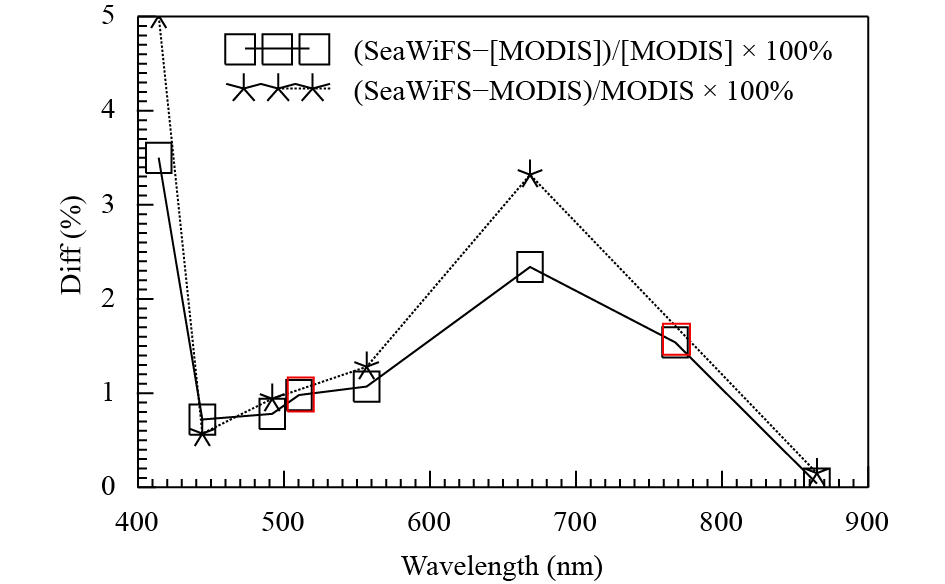Figure 4 The difference between the SeaWiFS and MODIS before and after SBA application.
3 The correction for the lunar observation geometry

Under an ideal condition, the two instruments take identical measurements when they view the same target at the same time. However, the same viewing and illumining geometries almost never occur in practice. To correct the impact of the lunar observation geometry, the “double difference” method is proposed to bridge the gap in the viewing geometry between the two instruments (Chander et al., 2013c). In this paper, another similar method called the “double-ratio” method is also proposed as a comparison.

The lunar irradiance changes with the lunar phase angle, which is related to the viewing and illumining geometry. The impact of the viewing and illumining geometry on the lunar cross-calibration should be taken into account. In fact, the ROLO model gives the irradiance pattern with the viewing and illumining geometry, which allows for its use as an intermediate reference to bridge the gap between the sensors.

3.1 “Double difference” method

The “double difference” is presented as

 $\Delta = \left({{I_{{\rm{ROLO}} - {\rm B},i}}-{I_{{\rm B},i}}} \right) - \left({{I_{{\rm{ROLO}} - {\rm A},i}}-{I_{{\rm A},i}}} \right),$ (7)

where ${I_{{\rm A},i}}$ is the observed lunar irradiance by satellite A at bandi, and ${I_{{\rm{ROLO}} - {\rm A},i}}$ is the simulated lunar irradiance for satellite A at bandi by the ROLO (311g version) model; ${I_{{\rm B},i}}$ is the observed lunar irradiance by satellite B at band i, and ${I_{{\rm{ROLO}} - {\rm B},i}}$ is the simulated lunar irradiance for satellite B at band i by the ROLO (311g version) model; $\Delta$ is the error of “double difference”.

The error analysis in Eq. (7) is shown as follows:

 ${I_{{A},i}} = {I_{{\rm A},i,{\rm{true}}}} + {I_{{\rm A},i,\,{\rm{true}}}}{\sigma _{{\rm A},i,{\rm{mea}}}}, \;\, \!\quad\quad\quad$ (8)
 ${I_{{\rm{ROLO}} - {\rm{A}},i}} = {I_{{\rm{A}},i,{\rm{true}}}} + {I_{{\rm{A}},i,{\rm{true}}}}{\sigma _{{\rm{A}},i,{\rm{ROLO}}}},$ (9)
 ${I_{{\rm{ROLO}} - {\rm B},i}} = {I_{{\rm B},i,{\rm{true}}}} + {I_{{\rm B},i,{\rm{true}}}}{\sigma _{{\rm B},i,{\rm{ROLO}}}}. \;$ (10)

Here, we assume that lunar irradiance ${I_{{\rm A},i}}$ as measured by satellite A has two parts：One is the true value of lunar irradiance ( ${I_{{\rm A},i,{\rm{true}}}}$ ) and another is the relative error ( ${\sigma _{{\rm{earth}}\_{\rm{based}},{\rm{mea}}}}$ ) from the measurement. The simulated lunar irradiance for satellite A by ROLO (311g version) model ${I_{{\rm{ROLO}} - {\rm{A}},i}}$ also has two parts. One is the true value of lunar irradiance ${I_{{\rm A},i,\,{\rm{true}}}}$ and another is the relative error ${\sigma _{{\rm{A}},i,{\rm{ROLO}}}}$ from the ROLO (311g version) model. This is also true for satellite B.

When Eqs. (8)–(10) are substituted into Eq. (7), we obtain the following equation:

 \begin{aligned} {I_{{\rm B},i}} + & \Delta = {I_{{\rm B},i,{\kern 1pt} {\text{true}}}} + {I_{{\rm B},i,{\kern 1pt} {\text{true}}}}{\sigma _{{\rm B},i,{\text{ROLO}}}} \\ & + {I_{{\rm A},i,{\kern 1pt} {\text{true}}}}\left( {{\sigma _{{\rm A},i,{\text{mea}}}} - {\sigma _{{\rm A},i,{\text{ROLO}}}}} \right). \end{aligned} (11)

As shown in Eq. (11), the term ( ${I_{{\rm B},i}} + \Delta$ ) is used for checking the calibration. The error ( ${I_{{\rm B},i}} + \Delta$ ) has two parts: One is the error in the ROLO model and another is the difference between the ROLO model error and the satellite-measured error by satellite A.

3.2 “Double ratio” method

The “double ratio” method used in the ROLO model is presented as follows:

 ${{\frac{{{I_{{\rm A},i}}}}{{{I_{{\rm{ROLO}} - {\rm A},i}}}}} / {\frac{{{I_{{\rm B},i}}}}{{{I_{{\rm{ROLO}} - {\rm B},i}}}}}} = \Delta,$ (12)

where $\Delta$ is the error of “double ratio”. After Eqs. (8)–(10) are substituted into Eq. (12), which will be as follows:

 \begin{aligned} {I_{{\rm B},i}} \cdot \Delta = & {I_{{\rm B},i,{\kern 1pt} {\text{true}}}}\left\{ {1{\text{ + }}\frac{{{\sigma _{{\rm B},i,{\text{ROLO}}}} - {\sigma _{{\rm A},i,{\text{ROLO}}}}}}{{1{\text{ + }}{\sigma _{{\rm A},i,{\text{ROLO}}}}}}{\text{ + }}{\sigma _{{\rm A},i,{\text{mea}}}}} \right. \\ & + \left. {\frac{{{\sigma _{{\rm B},i,{\text{ROLO}}}} - {\sigma _{{\rm A},i,{\text{ROLO}}}}}}{{1{\text{ + }}{\sigma _{{\rm A},i,{\text{ROLO}}}}}}{\sigma _{{\rm A},i,{\text{mea}}}}} \right\}, \end{aligned} (13)

where $\displaystyle\frac{{{\sigma _{{\rm B},i,{\rm{ROLO}}}}-{\sigma _{{\rm A},i,{\rm{ROLO}}}}}}{{1+{\sigma _{{\rm A},i,{\rm{ROLO}}}}}}{\sigma _{{\rm A},i,{\rm{mea}}}}$ is a second-order finite quantity, which can be ignored. Therefore, Eq. (13) will be simplified as

 \begin{aligned} {I_{{\rm B},i}} \cdot \Delta = {I_{{\rm B},i,{\kern 1pt} {\text{true}}}} + {I_{{\rm B},i,{\kern 1pt} {\text{true}}}}\left( {{\sigma _{{\rm A},i,{\text{mea}}}} + \frac{{{\sigma _{{\rm B},i,{\text{ROLO}}}} - {\sigma _{{\rm A},i,{\text{ROLO}}}}}}{{1 + {\sigma _{{\rm A},i,{\text{ROLO}}}}}}} \right), \end{aligned} (14)

as shown ${I_{{\rm B},i}} \cdot \Delta$ is made up of ${\sigma _{{\rm A},i,{\rm{mea}}}}$ and the last term within the brackets of Eq. (14).

The consistency of the ROLO (311g version) model is 1.00%–2.00%. If the lunar viewing and illuminating geometry between satellites A and B are closer, ${\sigma _{{\rm B},i,{\rm{ROLO}}}}$ and ${\sigma _{{\rm A},i,{\rm{ROLO}}}}$ in Eq. (14) are closer. Therefore, the last term within the brackets of Eq. (14) can be neglected. The error of the “double ratio” arises primarily from the satellite A measurement error.

3.3 Comparing the results from the double difference and the double ratio

Aqua MODIS is selected by the Global Space-based Inter-Calibration System (GSICS) operational community as the calibration reference sensor in cross-sensor calibration and calibration intercomparisons (Xiong et al., 2016). In this study, the MODIS lunar irradiance is selected to evaluate the double ratio and double difference methods. Specifically, the lunar irradiance was measured by MODIS at 2000-03-24T20:21:31Z from the lunar phase angle of 54.70° and was chosen as a radiometric reference while the lunar irradiances of the other 42 samples in the MODIS were used to evaluate the methods.

As shown in Table 2, the lunar phase angle ranges from 50.93° to 55.60°. In addition, the ROLO model and MT2009 are used as intermediate references. For the double ratio method used in the ROLO model, the minimum relative difference is 2.00% in band 9 and the maximum is 2.30% in band 8. The average relative difference is 2.20%. For the double difference method, the minimum difference is 2.20% in band 10 and the maximum is 2.50% in band 19. The average relative difference is 2.30%. When the MT2009 model is used, the error in the double ratio method is also less than the double difference method. The results show that the double ratio method performs better than double difference approach. This finding is consistent with the analysis from Eqs. (11) and (14) since the double difference error relies on the combined accuracy of the lunar model and the reference instrument while the double ratio error primarily results from the accuracy of the reference instrument. In addition, the double ratio and double-difference approaches within ROLO perform better than they do within MT2009 since the ROLO has better model accuracy

Table 2 The calibration difference between the double difference and double ratio
 MODIS band ROLO model MT2009 Difference (double difference; %) Difference (double ratio; %) Difference (double difference; %) Difference (double ratio; %) 1 2.163 ± 0.012 2.150 ± 0.012 4.101 ± 0.082 3.988 ± 0.065 2 2.195 ± 0.012 2.183 ± 0.013 4.120 ± 0.103 4.040 ± 0.074 3 2.209 ± 0.012 2.198 ± 0.013 5.413 ± 0.772 5.300 ± 0.823 4 2.208 ± 0.013 2.182 ± 0.013 4.876 ± 0.523 3.549 ± 0.413 8 2.368 ± 0.014 2.290 ± 0.013 5.618 ± 0.154 4.998 ± 0.080 9 2.194 ± 0.012 2.000 ± 0.012 5.351 ± 0.132 5.002 ± 0.992 10 2.167 ± 0.012 2.141 ± 0.013 4.128 ± 0.922 3.473 ± 0.847 11 2.237 ± 0.014 2.208 ± 0.014 4.057 ± 0.744 3.216 ± 0.649 12 2.197 ± 0.013 2.151 ± 0.013 4.124 ± 0.853 3.240 ± 0.745 17 2.362 ± 0.016 2.197 ± 0.012 4.980 ± 0.976 4.677 ± 0.823 18 2.433 ± 0.017 2.160 ± 0.011 4.774 ± 0.904 4.549 ± 0.671 19 2.465 ± 0.017 2.154 ± 0.012 4.143 ± 0.077 3.786 ± 0.062

To verify the double ratio analytics mentioned above, we used the double ratio method to deliver the MODIS lunar irradiance as measured at phase angles from 54.70° ${I_{{\rm{MODIS}},i,{{54.696}^\circ}}}$ to 54.41° and 50.92°. The double-ratio, ${I'_{{\rm{MODIS}},i,{\rm{angle}}}}$ , is expressed as:

 ${I'_{{\rm{MODIS}},i,{\rm{angle}}}} = \frac{{{I_{{\rm{MODIS}},i,{{54.70}^\circ}}}}}{{{I_{{\rm{ROLO}},i,{{54.70}^\circ}}}}}{I_{{\rm{ROLO}},i,{\rm{angle}}}},$ (15)

where ${I_{{\rm{ROLO}},i,{{54.70}^\circ}}}$ is the lunar irradiance simulated by the ROLO model (phase angle = 54.70°). Is ${I_{{\rm{ROLO}},i,{\rm{angle}}}}$ also the lunar irradiance simulated by the ROLO model ( ${\rm{{\rm{angle}} = }}{54.41^\circ}\;{\rm{or}}\; {50.92^\circ}$ )?

As shown in Fig. 5, the blue line is the difference between the double ratio result and the lunar irradiance in MODIS (phase angle = 54.41°). The red line is also the difference between the double ratio result and the lunar irradiance of MODIS (phase angle = 50.92°); and the black line is the difference between the double ratio result and average of lunar irradiance in MODIS for 42 samples (average lunar angle = 52.41°). The radiometric reference of the MODIS was measured at 54.70°. Thus, the lunar phase between the radiometric reference and the measurement is closer, indicating the difference between the double ratio result and the measurement.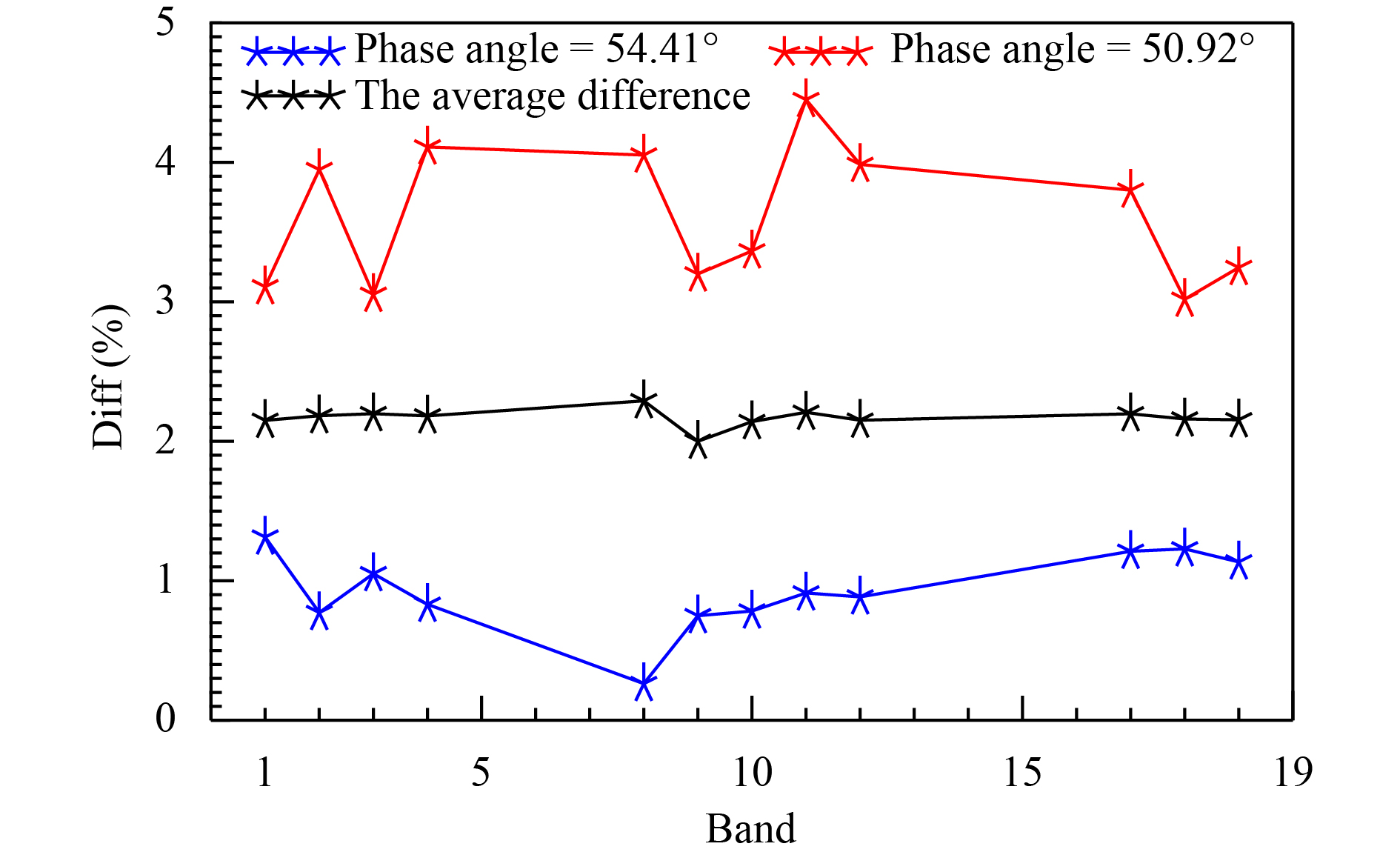Figure 5 The difference in the lunar irradiance between the double ratio result and the MODIS measurement.
4 Cross-calibration between sensors

The two methods provides us with a new way to cross-calibrate the sensors with different RSRs and to view the illumining geometry of the moon. Here, the lunar irradiance of the MODIS as measured at 2000-03-24 T20:21:31Z (lunar phase = 54.70°) is selected as a radiometric reference to check the double ratio and SBA approaches used together.

4.1 SeaWiFS with MODIS

SeaWiFS and MODIS instrument agree at the 3%–8% level (Eplee et al., 2011). As shown in Fig. 1 and Table 1, some MODIS bands and SeaWiFS bands are very close, but the RSRs of them are different, and the MODIS bands do not cover SeaWiFS bands 4 and 7 (Eplee et al., 2011). Moreover, the lunar phase between the MODIS measurements and the SeaWiFS measurements are not the same. All of those problems can be solved by using the SBA and double ratio method.

As shown in Fig. 6, the RSRs and the observation geometry between the SeaWiFS and MODIS were rectified. The moon’s smooth reflectance can help us to transfer the MODIS measurements to bands 2 and 4 of the SeaWiFS. The ROLO model helps us to transfer the MODIS measurements to SeaWiFS at lunar phase angles of 54.49° and 32.00°. For the SeaWiFS measured at 54.49°, the mean difference between the SeaWiFS and ROLO model is 4.07%, and the SeaWiFS and “MODIS” (the result of double ratio and SBA) is 2.72%; at 23.00°, the mean difference between the SeaWiFS and ROLO models is 5.10%, and the difference between the MODIS and SeaWiFS is 1.48%. It is shown that the combination of the double ratio and SBA methods is consistent with the accuracy of the SeaWiFS (2%–3%), which is better than the results from the ROLO model alone.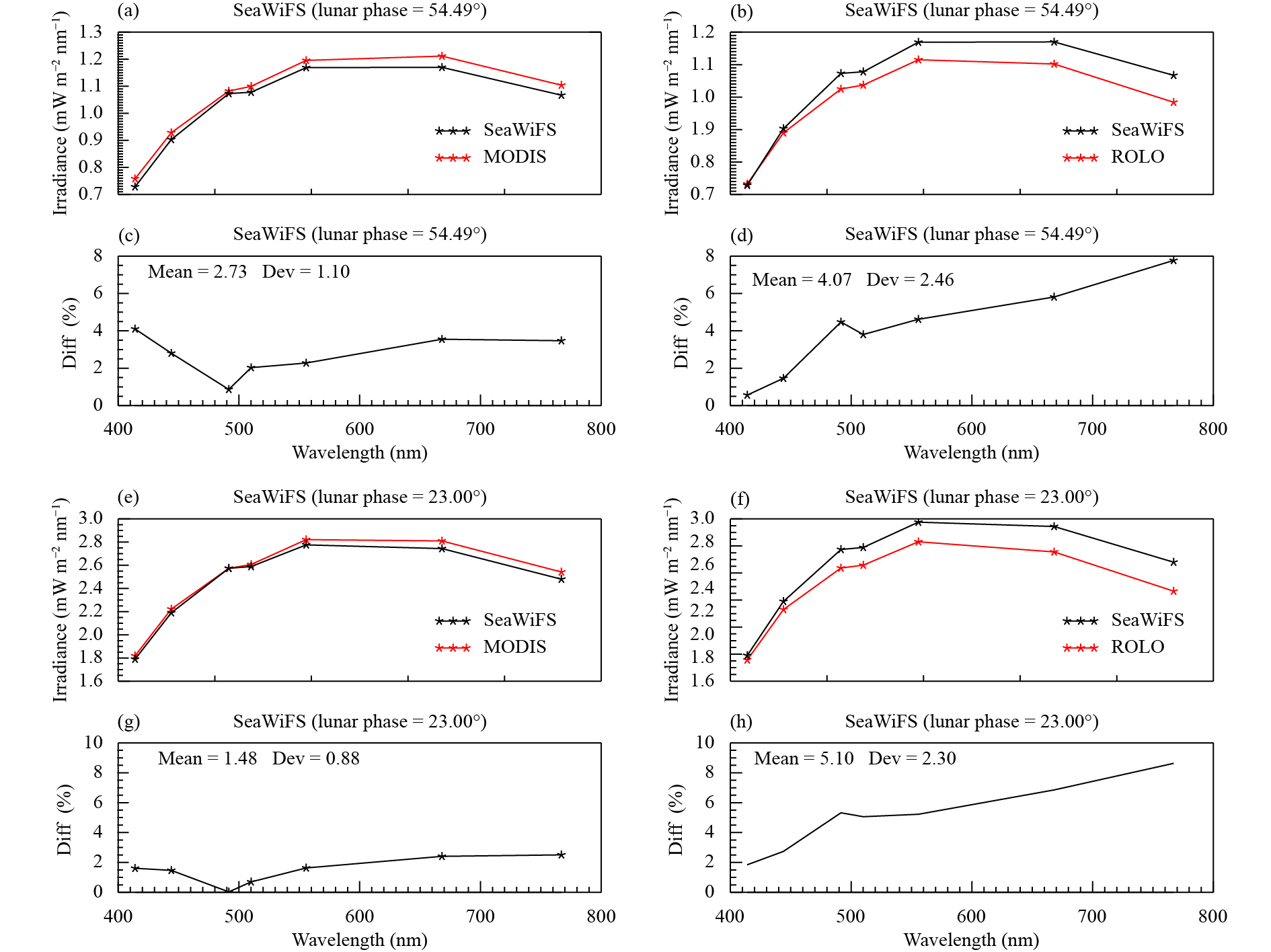Comparisons (a, c, e, g) between ROLO and SeaWiFS and (b, d, f, h) between SeaWiFS and MODIS. (c, d) Diff = [(ROLO – SeaWiFS)/SeaWiFS × 100%] and (g, h) Diff = [(MODIS – SeaWiFS)/SeaWiFS × 100%]. Figure 6 The cross-calibration between the SeaWiFS and MODIS. The SeaWiFS lunar phase = (a–d) 54.49° and (e–h) 32.00°
4.2 ALI with MODIS

The ALI is an imager onboard the Earth Observing Mission 1 (EO-1) satellite. ALI is consistent with MODIS to within 5.0% according to near-simultaneous desert observations (Chander et al., 2013b). The results of the SBA and double ratio approach used in the cross-calibration between the ALI and “MODIS” are shown in Fig. 7. It is found that the mean relative difference between the ROLO and the ALI is larger than the difference between the “MODIS” and ALI, and the mean relative difference between the ALI and “MODIS” is less than 5.0%.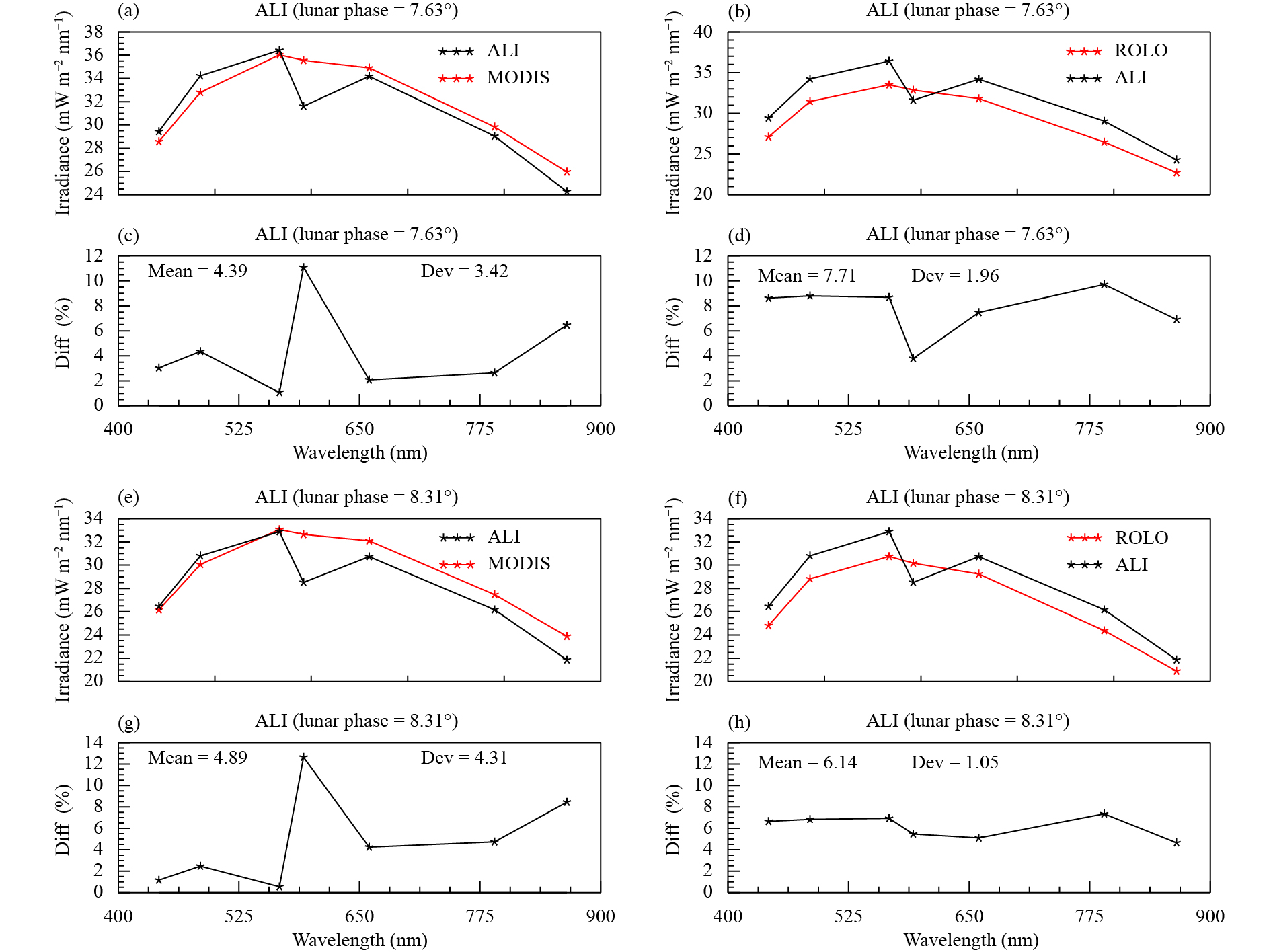Comparisons (a, c, e, g) between ROLO and ALI, and (b, d, f, h) between ALI and MODIS. (c, d) Diff = [(ROLO – ALI)/ALI × 100%] and (g, h) Diff = [(MODIS – ALI)/ALI × 100%]. Figure 7 The cross-calibration between ALI and MODIS. The lunar phase = (a–d) 7.63° and (e–h) 8.31°
5 Summary

Inconsistent RSRs and the difference in lunar observation geometry can have a great effect on the cross-calibration of different sensors and their long-term characterization. When comparing the double difference and double ratio, the uncertainty of the benchmark instrument is less than that of the intermediate reference, and the intermediate reference has a good consistency; the double ratio method is thus better than the double difference approach. When the uncertainty of the intermediate reference is large, the double difference can amplify the uncertainty of the cross-calibration, and the double ratio performs better. Moreover, the lunar viewing and illumining geometry is closer, and the double ratio error becomes smaller. In general, the double ratio performs better than the double difference.

Since the development of the MEdium Resolution Spectral Imager (MERSI) onFY-3C, the space view (SV) field angle is increased to acquire full-disk moon images (Wu et al., 2016; Chen et al., 2018). This was the first time that the Chinese earth observation satellite could capture full-disk moon images. The function of the lunar view is enhanced for the MERSI on FY-3D. These lunar-based cross-calibrations will be made available for the Chinese earth observation satellite. The method in this study could be applied to MERSI onFY-3C and FY-3Dto improve their data quality, which will be explored in our future studies.

Acknowledgments. The authors would like to acknowledge Dr. Tom Stone for making their ROLO lunar irradiance models publicly available and D. Y. Wang for providing the lunar irradiance of the satellite. We also thank the MODIS and SeaWiFS groups for freely providing their high-quality data.

References